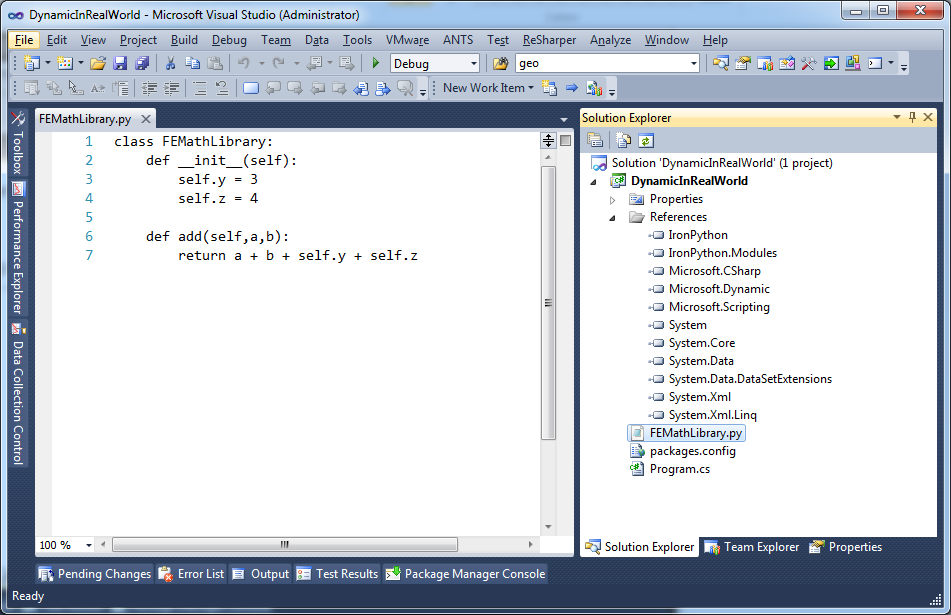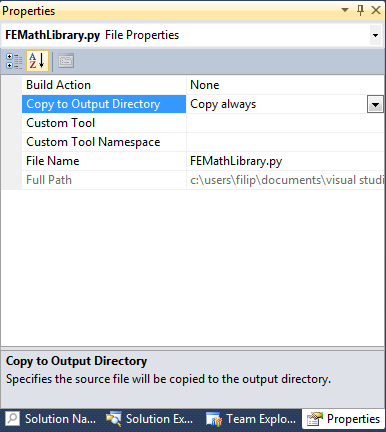# Using dynamic in the real world with IronPython

## Posted by Filip Ekberg on 04 Oct 2011

My last posts have been a lot about what you can do to explore the dynamic world in .NET and what it might be good for. But I think it's time for some real fun with dynamic!

So go ahead and fire up Visual Studio, see to it that you got NuGet installed, now let's get rocking!

The first thing you want to do is to create a new console application with any name of your choice, then I want you to go into:

``````Tools -> Library Package Manager -> Package Manager Console
``````

This is the NuGet console, here you can do all kinds of neat stuff, we are going to use it to install IronPython into our project, so simply write the following in the console:

``````Install-Package IronPython
``````

After 30 seconds to a minute or so, you should see something like this:

``````PM> Install-Package IronPython
Successfully installed 'IronPython 2.6.1'.
Successfully added 'IronPython 2.6.1' to DynamicInRealWorld.
``````

This means that IronPython was successfully added to our project! But wait a minute, what is IronPython? This is the definition of it according to Wikipedia:

IronPython is an implementation of the Python programming language targeting the .NET Framework and Mono.

A couple of lines below that something interesting is stated, have a look at this part:

IronPython is implemented on top of the Dynamic Language Runtime (DLR), a library running on top of the Common Language Infrastructure that provides dynamic typing and dynamic method dispatch, among other things, for dynamic languages. The DLR is part of the .NET Framework 4.0 and is also a part of trunk builds of Mono. The DLR can also be used as a library on older CLI implementations.

Let's take a step back and think about what this means, basically it means that we can write Python code and have it interact with our code that we write in .NET! Let's look at some code and see if this clears up a bit.

I've got a Python library that is somewhat going to be used for advance math-calculations and it looks like this:

``````class FEMathLibrary:
def __init__(self):
self.y = 3
self.z = 4

return a + b + self.y + self.z
``````

For those that don't know Python programming, what this basically does is that it creates a class, gives it two properties ( y and z ) and then it has a method called add that takes two parameters when it's called ( a and b ). Now, I've spent years and years optimizing this very advance mathematical operation and I don't want to re-write it in .NET, that would take a lot of time and cost to much. It's also worth mentioning that Python is a dynamically typed programming language and c# is a statically typed programming language.

I imported this file into my visual studio project and the project should now look somewhat like this:You also need to change the property on the file that states that it will be copied to the output directory, the properties for the file should look like this:Let's dig into the C# code, shall we?

This is what I want to do ( in human understandable terms ):

• Create a runtime that can run Python code
• Tell the runtime to run my file
• Create an instance of FEMathLibrary
• Print the result

The only two things that you need to import are the following: using System; using IronPython.Hosting;

IronPython.Hosting contains a lot of classes that we can use to help us achieve the above. One of these things being a class called `Python` that has a very interesting method on it called `CreateRuntime`, we can use that like this to actually create our runtime:

``````var runtime = Python.CreateRuntime();
``````

The second thing on the list is to tell the runtime to load the file, this is done by using the method `UseFile` on the runtime-instance. We should now have something that looks pretty much like this:

``````var runtime = Python.CreateRuntime();
dynamic source = runtime.UseFile("FEMathLibrary.py");
``````

Here's what's interesting, the source variable is dynamic! If this was python, we could write something like this:

``````instance=FEMathLibrary()
print(result)
``````

So what about the C# version? How do we create the instance of FEMathLibrary? Our dynamic object is what will let us do this, we simply write the following to create an instance of FEMathLibrary:

``````var instance = source.FEMathLibrary();
``````

The rest is pretty much the same thing! Here's how it turned out:

``````var runtime = Python.CreateRuntime();
dynamic source = runtime.UseFile("FEMathLibrary.py");

dynamic instance = source.FEMathLibrary();